Python | Pandas Index.identical()

• Last Updated : 17 Dec, 2018

Python is a great language for doing data analysis, primarily because of the fantastic ecosystem of data-centric python packages. Pandas is one of those packages and makes importing and analyzing data much easier.

Pandas Index.identical() function determine if two Index objects contains the same elements. If they contain the same elements then the function returns True else the function returns False indicating the values contained in both the Indexes are different. This is similar to the Index.equals(), but check that other comparable attributes are also equal.

Syntax: Index.identical(other)

Parameters :
Other : index

Returns : boolean value

Example #1: Use Index.identical() function to check if two Indexes contain same elements and if other attributes are identical or not.

 # importing pandas as pdimport pandas as pd  # Creating the first Indexidx1 = pd.Index(['Labrador', 'Beagle', 'Labrador', 'Lhasa', 'Husky', 'Beagle'])  # Creating the second Indexidx2 = pd.Index(['Labrador', 'Beagle', 'Pug', 'Lhasa', 'Husky', 'Pitbull'])  # Print the first and second Indexprint(idx1, '\n', idx2)

Output :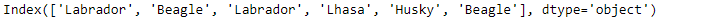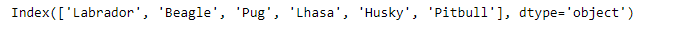Let’s check if the two Indexes are identical or not.

 # Checking the equality of the two Indexesidx1.identical(idx2)

Output :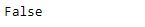As we can see in the output, the Index.identical() function has returned False indicating that the Indexes are not equal.

Example #2: Use Index.identical() function to check if the input indexes are identical to each other or not.

 # importing pandas as pdimport pandas as pd  # Creating the first Indexidx1 = pd.Index(['Jan', 'Feb', 'Mar', 'Apr', 'May', 'Jun',                'Jul', 'Aug', 'Sep', 'Oct', 'Nov', 'Dec'])  # Creating the second Indexidx2 = pd.Index(['Jan', 'Feb', 'Mar', 'Apr', 'May', 'Jun',                'Jul', 'Aug', 'Sep', 'Oct', 'Nov', 'Dec'])  # Print the first and second Indexprint(idx1, '\n', idx2)

Output :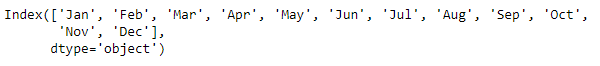Let’s check if the two Indices are identical to each other or not.

 # test the equalityidx1.identical(idx2)

Output :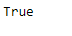The function has returned True indicating that both the Indexes are identical to each other.

My Personal Notes arrow_drop_up